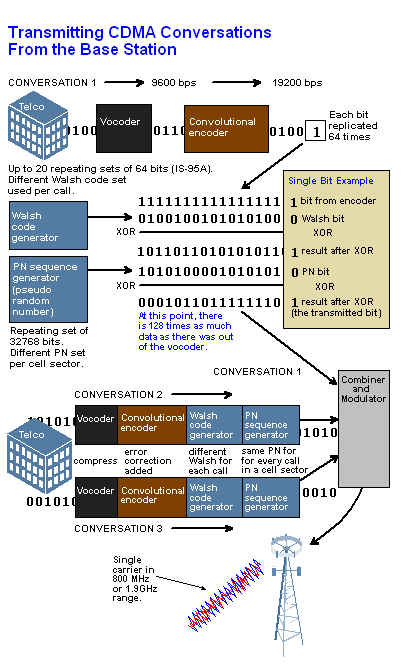# Viterbi algorithm

(redirected from Forward algorithm)

## Viterbi algorithm

[vi¦ter·bē ′al·gə‚rith·əm]
(communications)
A decoding procedure for convolutional codes that uses the maximum-likelihood method.
McGraw-Hill Dictionary of Scientific & Technical Terms, 6E, Copyright © 2003 by The McGraw-Hill Companies, Inc.

## Viterbi decoder

A decoding algorithm developed in the late 1960s by Andrew Viterbi that is used to decode a particular convolutional code. Viterbi decoders have been the most effective way to decode wireless voice communications in satellite and cellphone transmissions. Viterbi outputs a 0 or a 1 based on its estimate of the input bit. See CDMA, convolutional code and turbo code.

Using the Viterbi DecoderIn the CDMA technology, the Viterbi decoder in the cellphone is used to correct the errors in the convolutional code that was generated by the base station. See CDMA.
Copyright © 1981-2019 by The Computer Language Company Inc. All Rights reserved. THIS DEFINITION IS FOR PERSONAL USE ONLY. All other reproduction is strictly prohibited without permission from the publisher.
Mentioned in ?
References in periodicals archive ?
In Section 3, we first review the high-order transformation algorithm proposed by Hadar and Messer  and then we introduce EOTFA, an entropy-based order-transformation forward algorithm for computing the optimal state sequence for any generalized HHMM.
In this section, we introduce an efficient entropy-based algorithm that used reduction approach, namely, entropy-based order-transformation forward algorithm (EOTFA) to compute the optimal state sequence based on entropy of any generalized HHMM.
This binary observation function not only cancels the set of visible locations but also eliminates every path that would have passed by these positions at this particular time, due to the recursive nature of the forward algorithm. We will explain this in detail in the next subsection.
This probability is denoted by [P.sub.t]([s.sub.i]) and is calculated using the forward algorithm:
Initially introduced in speech recognition problems, the HMM Forward algorithm has become increasingly popular in bioinformatics.
Then we recognize the alert belonging to attack scenarios with the Forward algorithm of HMM.
4 one can note that the proposed algorithm provides a larger bandwidth when compared to the method proposed in  and to the forward algorithm .
Complexity analysis Parameter Number of multiplication Complexity of forward algorithm NTK(M2+D) Complexity of backward algorithm NTK(M2+D) Complexity of Baum-Welch algorithm NTKM2D Table 2.
Since we trace back the forward algorithm, we start initially with N=[2.sup.p], with p = 1, 2, 3, ..., n.
Both forward algorithms and backward algorithms have certain shortcomings on industrial data sets.

Site: Follow: Share:
Open / Close